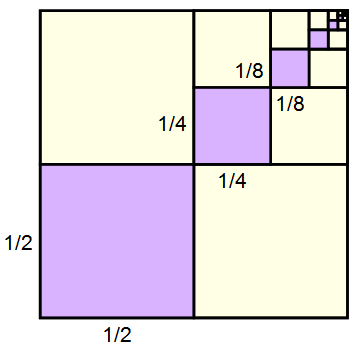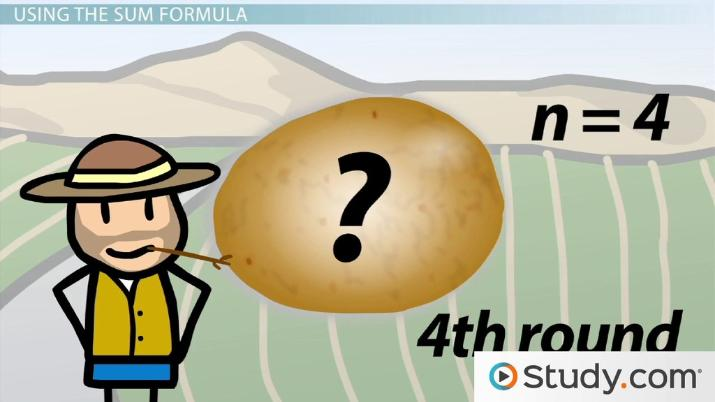# Finding the Sum of a Geometric Sequence

Thomas Coleman, Yuanxin (Amy) Yang Alcocer, Robert Ferdinand
• Author
Thomas Coleman

Thomas has experience as both a tutor and instructor, in formal settings at the University of South Florida and tutoring centers, and informally with individual students. He has a master's degree in mathematics from the University of South Florida.

• Instructor
Yuanxin (Amy) Yang Alcocer

Amy has a master's degree in secondary education and has been teaching math for over 9 years. Amy has worked with students at all levels from those with special needs to those that are gifted.

• Expert Contributor
Robert Ferdinand

Robert Ferdinand has taught university-level mathematics, statistics and computer science from freshmen to senior level. Robert has a PhD in Applied Mathematics.

Learn how to find the sum of a geometric sequence and the formula used. See examples of geometric sum equations and how to find the sum of a geometric series. Updated: 08/26/2021

Show

## What is a Geometric Series

A few important building blocks are necessary before working with geometric series. The first is the mathematical sequence which is simply a set of numbers with a defined order. A specific case which we will use to construct geometric series is the geometric sequence: a sequence in which each term is generated by multiplying the previous term by a fixed number called the common ratio. Any geometric sequence is defined completely by its first term and its common ratio. For instance, the sequence

$$1,2,4,8,16,\dots$$

is geometric, as each term is obtained by multiplying the previous one by a constant; in this case, the constant is 2. Note the use of the word "constant": the number by which each term is multiplied is unchanged throughout the sequence. The sequence

$$2,4,16,256\dots$$

however, is not geometric. Note that each term is the square of the one that precedes it - that is, each term is multiplied by itself, not the same number! This sequence is well defined, but it is NOT geometric.

The ability to find any term of a sequence can be a useful tool. (Here, n is indeed a variable, meaning that any particular term can be found: the 1st, 2nd, 3rd, 4th, et cetera.) The nth term will be denoted {eq}a_n {/eq}.

What is already known about this number? As each term is obtained from the previous one, it is the previous term multiplied by the common ratio, which will be denoted r. That is,

$$a_n=a_{n-1}r$$

But this number is just the previous term multiplied by r, and that previous term is just the term before it multiplied by r, and this process continues until the first term is reached. This term is usually denoted {eq}a_0 {/eq}. (Note that the subscript is zero, not one. This may seem confusing at first, but will simplify notation greatly in the future.) Therefore,

$$a_n=a_{n-1}r=a_{n-2}r^2=\cdots=a_0r^n$$

Consider the geometric sequence {eq}3,6,12,24,\dots {/eq}. What is the 10th term? Note well: the 10th term will be {eq}a_9 {/eq}, as the first term is {eq}a_0 {/eq}. With the formula above, finding it is simple once the first term and common ratio are known. The first term is simply 3; divide any term by the term before it to find the common ratio. {eq}r=6\div3=2 {/eq}. (Checking that other terms have the same ratio verifies that the sequence is indeed geometric.) The 10th term follows immediately:

$$a_9=a_0r^9=3\cdot2^9=3\cdot512=1536$$

Writing out the sequence step by step bears out the results of the formula:

$$a_0=3\\ a_1=3\cdot2=6\\ a_2=6\cdot2=12\\ a_3=12\cdot2=24\\ a_4=24\cdot2=48\\ a_5=48\cdot2=96\\ a_6=96\cdot2=192\\ a_7=192\cdot2=384\\ a_8=384\cdot2=768\\ a_9=768\cdot2=1536$$Now the question of "geometric series" can be tackled. A series is the sum of the terms of a sequence; a geometric series therefore, is the sum of the terms of a geometric sequence. For a geometric sequence {eq}a_n=a_0r^n {/eq}, the geometric series of this sequence is written

$$\sum_{i=0}^{n-1}a_0r^i$$

This "sigma notation" (the large symbol is the capital Greek letter sigma) is used as shorthand for sums. It expands to

$$a_0r^0+a_0r^1+\cdots+a_0r^{(n-1)}$$

The variable below the sigma (called the index) is replaced by 1 for the first term, 2 for the second, and so on until the number above the sigma is reached. In this case, that number is {eq}n-1 {/eq}, but if a constant is in its place, the sum is a finite sum that ends when the index reaches that number.

For instance, consider the geometric series {eq}a_n=3\cdot2^n {/eq}, which has first term 3 and common ratio 2. Its series is simply adding the terms together. In order to find the third term in the series (not the sequence), the first three terms are added together. The notation for this sum looks like this:

$$\sum_{i=0}^23\cdot2^i=3\cdot2^0+3\cdot2^1+3\cdot2^2=3\cdot1+3\cdot2+3\cdot4=3+6+12=21$$An error occurred trying to load this video.

Try refreshing the page, or contact customer support.

Coming up next: Understand the Formula for Infinite Geometric Series

### You're on a roll. Keep up the good work!

Replay
Your next lesson will play in 10 seconds
• 0:01 A Geometric Sequence
• 1:21 The Sum Formula
• 2:18 Using the Sum Formula
• 3:19 Another Example
• 4:11 Lesson Summary
Save Save

Want to watch this again later?

Timeline
Autoplay
Autoplay
Speed Speed

## Sum Formula of Geometric Series:

Earlier in the lesson, a simpler shorthand for the {eq}n {/eq}th term of a geometric sequence was described. The same can be done for a geometric series, with a little reasoning. First, for convenience, use {eq}S_n {/eq} to denote the sum of the terms from 0 to {eq}n {/eq}:

$$S_n=\sum_{i=0}^na_0r^i$$

Proceed with a little lateral thinking: notice that each term contains {eq}r {/eq}, except for the first term. That problem can be eliminated by multiplying the entire series by {eq}r {/eq}:

$$rS_n=r\sum_{i=0}^na_0r^i=r(a_0+a_0r+\cdots+a_0r^n)=a_0r+a_0r^2+\cdots+a_0r^{(n+1)}=\sum_{i=0}^na_0r^{(i+1)}$$

And what happens when these two series are subtracted?

$$S_n-rS_n=\sum_{i=0}^na_0r^i-\sum_{i=0}^na_0r^{(i+1)}=(a_0+a_0r+\cdots+a_0r^n)-(a_0r+a_0r^2+\cdots+a_0r^{(n+1)})$$

But wait. The second term of the first sum is the same as the first term of the second sum. Indeed, this pattern continues: the third term of the first sum is the same as the second term of the second sum, and so on. Rearrange the terms to align these identical terms together.

$$S_n-rS_n=a_0+a_0r-a_0r+a_0r^2-a_0r^2+\cdots+a_0r^n-a_0r^n-a_0r^{(n+1)}$$

These terms cancel in pairs, leaving only the last term from the first sum and the first term from the second sum.

$$S_n-rS_n=a_0-a_0r^n=a_0(1-r^{(n+1)})$$

Solve for {eq}S_n {/eq}.

$$S_n-rS_n=a_0(1-r^{(n+1)})\\ S_n(1-r)=a_0(1-r^{(n+1)})\\ S_n=a_0\frac{(1-r^{(n+1)})}{(1-r)}$$

### Sum of Infinite Geometric Series:

Now, an interesting question arises. Given any well-defined series, finding the sum of any number of its terms is a matter of calculating each term and adding them all together - provided that the number of terms is finite. What if there are infinitely many terms? Can such a sum be calculated? Does it even exist?

The answer depends upon the series. Only geometric series are considered for this lesson, for which there is a very clear answer. The formula for a sum of a finite number of terms - a partial sum - has already been defined above. Then, for an infinite series, this partial sum tends towards infinite terms.

$$\sum_{i=0}^\infty a_0r^i=\lim_{n\to\infty}S_n=\lim_{n\to\infty}a_0\frac{(1-r^{(n+1)})}{(1-r)}$$

To unlock this lesson you must be a Study.com Member.

• Activities
• FAQs

## Sum of First n Terms of Geometric Sequence: Practice Problems

#### Key Terms

• Geometric Sequence: A sequence in which each term is the previous term, multiplied by a fixed constant called the common ratio r.
• Sum of Geometric Sequence: The number we get when we add the first n terms of a Geometric Sequence

• Paper
• Pencil
• Calculator

#### Practice Questions (Show All Your Work):

(a) Find the sum of the first 6 terms of the following geometric sequence:

-3, 6, -12, 24,...

(b) Find the sum of the first 12 terms of the following geometric sequence:

8, 4, 2, 1,...

(a) Step 1: To find the sum we identify the following:

First term a = -3

The common ratio, r = -2 (each term is the previous term multiplied by -2)

The number of terms, n = 6.

Step 2: Apply the sum of Geometric Sequence formula to the information in Step 1 to get:

S = a(r^n - 1)/(r - 1) = -3((-2)^6 - 1)/(-2 - 1) = -3(63)/(-3) = 63.

(b) Step 1: To find the sum we identify the following:

The first term, a = 8

The common ratio, r = 1/2 = 0.5 (each term is the previous term multiplied by 1/2)

The number of terms, n = 12.

Step 2: Apply the sum of Geometric Sequence formula to the information in Step 1 to get:

S = a(r^n - 1)/(r - 1) = 8((0.5)^(12) - 1)/(0.5 - 1) = 8(-0.9997)/(-0.5) = 15.9960.

#### What is the sum of geometric progression?

A geometric sequence (or geometric progression) is a sequence of numbers in which each one is the product of the previous number and a constant, known as the common ratio. A sum of a geometric progression is the sum of a given number of terms from the sequence, in order.

#### What is sum of geometric series?

A series is the sum of the terms of a well defined sequence. A geometric sequence is one in which each term is the product of the previous term and a given constant, and a geometric series is a series based on a geometric sequence.

#### What is the formula for the sum of infinite geometric series?

Where a is the initial value and r is the common ratio: a * (1/(1 - r))

Note that this formula only applies if |r|<1! If this is not the case, then the series diverges.

#### What is the formula of sum of GP?

Where a is the initial value and r is the common ratio:

a * (1 - r^(n+1))/(1 - r)

Remember that to find a given term, n should be one less than the number of that term, as the index begins at 0. For instance, to find the 10th term, n=9.

### Register to view this lesson

Are you a student or a teacher?